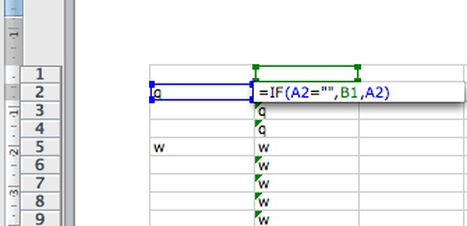Why Do Excel Formulas Begin With an Equal Sign

Offbeat — 25 February 2014Those of you who work regularly on the Excel program would know the way to enter formulas in cells on an Excel sheet. You may have noticed that every entered formula needs to be started with an equal (=) sign. Have you wondered about the reason why an Excel formula always begins with an equal sign? If yes, then here is the solution to your curious thought.

An equal sign in a cell indicates to Excel that you are not going to enter any text in that particular cell. It tells Excel to interpret that entry as a formula with numbers mentioned afterward. You may have noticed that adding another character like an apostrophe before the equal sign nullifies this effect. When this effect is nullified, Excel no longer interprets your input as a formula but as some text. Thus, you always need to start a formula entry with an equal sign. This is the default understanding of the Excel program.

In fact, people choose to replace an equal sign with another character when they do not intend to enter a formula in a cell. Replacing this sign means that your entry will be treated as text even though you have mentioned numbers afterward. Displaying an equal sign as your first character indicates that your given entry is equal to the mentioned formula. Thus, keeping various inputs on a standard platform makes it easier for Excel to perform its functions smoothly. That is the reason every formula needs to begin with an equal sign, which is a standard functionality feature for the Excel program.

Thus, equal means ‘equivalent to’ when you talk of its mathematical interpretation. By using this sign, you are simply telling Excel what your entry is equivalent to. Indication of an equal sign always displays a formula in Excel.